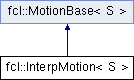FCL  0.6.0 Flexible Collision Library
fcl::InterpMotion< S > Class Template Reference

Linear interpolation motion Each Motion is assumed to have constant linear velocity and angular velocity The motion is R(t)(p - p_ref) + p_ref + T(t) Therefore, R(0) = R0, R(1) = R1 T(0) = T0 + R0 p_ref - p_ref T(1) = T1 + R1 p_ref - p_ref. More...

`#include <interp_motion.h>`

Inheritance diagram for fcl::InterpMotion< S >:## Public Member Functions

InterpMotion ()
Default transformations are all identities.

InterpMotion (const Matrix3< S > &R1, const Vector3< S > &T1, const Matrix3< S > &R2, const Vector3< S > &T2)
Construct motion from the initial rotation/translation and goal rotation/translation.

InterpMotion (const Transform3< S > &tf1_, const Transform3< S > &tf2_)

InterpMotion (const Matrix3< S > &R1, const Vector3< S > &T1, const Matrix3< S > &R2, const Vector3< S > &T2, const Vector3< S > &O)
Construct motion from the initial rotation/translation and goal rotation/translation related to some rotation center.

InterpMotion (const Transform3< S > &tf1_, const Transform3< S > &tf2_, const Vector3< S > &O)

bool integrate (double dt) const
Integrate the motion from 0 to dt We compute the current transformation from zero point instead of from last integrate time, for precision.

computeMotionBound (const BVMotionBoundVisitor< S > &mb_visitor) const
Compute the motion bound for a bounding volume along a given direction n, which is defined in the visitor.

computeMotionBound (const TriangleMotionBoundVisitor< S > &mb_visitor) const
Compute the motion bound for a triangle along a given direction n, which is defined in the visitor.

void getCurrentTransform (Transform3< S > &tf_) const
Get the rotation and translation in current step.

void getTaylorModel (TMatrix3< S > &tm, TVector3< S > &tv) const

const Vector3< S > & getReferencePoint () const

const Vector3< S > & getAngularAxis () const

getAngularVelocity () const

const Vector3< S > & getLinearVelocity () constPublic Member Functions inherited from fcl::MotionBase< S >
virtual bool integrate (S dt) const =0
Integrate the motion from 0 to dt.

void getCurrentTransform (Matrix3< S > &R, Vector3< S > &T) const
Get the rotation and translation in current step.

void getCurrentTransform (Quaternion< S > &Q, Vector3< S > &T) const

void getCurrentRotation (Matrix3< S > &R) const

void getCurrentRotation (Quaternion< S > &Q) const

void getCurrentTranslation (Vector3< S > &T) const

const std::shared_ptr< TimeInterval< S > > & getTimeInterval () const

## Protected Member Functions

void computeVelocity ()

Quaternion< S > deltaRotation (S dt) const

Quaternion< S > absoluteRotation (S dt) const

## Protected Attributes

Transform3< S > tf1
The transformation at time 0.

Transform3< S > tf2
The transformation at time 1.

Transform3< S > tf
The transformation at current time t.

Vector3< S > linear_vel
Linear velocity.

angular_vel
Angular speed.

Vector3< S > angular_axis
Angular velocity axis.

Vector3< S > reference_p
Reference point for the motion (in the object's local frame)Protected Attributes inherited from fcl::MotionBase< S >
std::shared_ptr< TimeInterval< S > > time_interval_

## Detailed Description

### template<typename S> class fcl::InterpMotion< S >

Linear interpolation motion Each Motion is assumed to have constant linear velocity and angular velocity The motion is R(t)(p - p_ref) + p_ref + T(t) Therefore, R(0) = R0, R(1) = R1 T(0) = T0 + R0 p_ref - p_ref T(1) = T1 + R1 p_ref - p_ref.

The documentation for this class was generated from the following files: